## Velocity Field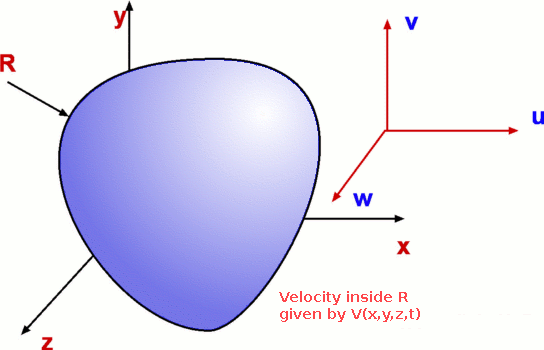Figure 3.1 : Velocity Field

Velocity field implies a distribution of velocity in a given region say R (Fig.3.1). It is denoted in a functional form as V(x,y,z,t) meaning that velocity is a function of the spatial and time coordinates. It is useful to recall that we are studying fluid flow under the Continuum Hypothesis which allows us to define velocity at a point. Further velocity is a vector quantity i.e., it has a direction along with a magnitude. This is indicated by writing velocity field as(3.1)

Velocity may have three components, one in each direction, i.e, u,v and w in x, y and z directions respectively. It is usual to write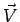as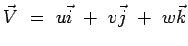(3.2)

It is clear that each of u,v and w can be functions of x,y,z and t. Thus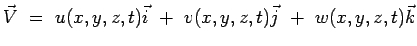(3.3)

Each of the other variables involved in a fluid flow can also be given a field representation. We have temperature field, T(x,y,z,t), pressure field, p(x,y,z,t), density field,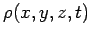etc.

(c) Aerospace, Mechanical & Mechatronic Engg. 2005
University of Sydney